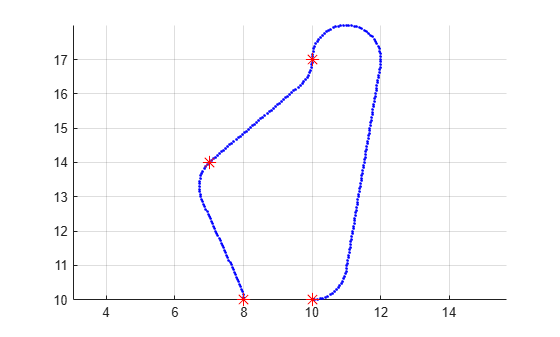# append

Add states to end of path

## Syntax

``append(path,states)``

## Description

example

````append(path,states)` appends the state samples, `states`, to the end of the `path`.```

## Examples

collapse all

Create a navPath object based on multiple waypoints in a Dubins space.

`dubinsSpace = stateSpaceDubins([0 25; 0 25; -pi pi])`
```dubinsSpace = stateSpaceDubins with properties: SE2 Properties Name: 'SE2 Dubins' StateBounds: [3×2 double] NumStateVariables: 3 Dubins Vehicle Properties MinTurningRadius: 1 ```
`pathobj = navPath(dubinsSpace)`
```pathobj = navPath with properties: StateSpace: [1×1 stateSpaceDubins] States: [0×3 double] NumStates: 0 ```
```waypoints = [... 8 10 pi/2; 10 12 pi/4; 12 17 pi/2; 11 10 -pi]; append(pathobj, waypoints);```

Interpolate that path so that it contains exactly 250 points.

`interpolate(pathobj, 250)`

Visualize the interpolated path and the original waypoints.

```figure; grid on; axis equal; hold on; plot(pathobj.States(:,1), pathobj.States(:,2), ".b"); plot(waypoints(:,1), waypoints(:,2), "*r", "MarkerSize", 10)```Calculate length of path.

```len = pathLength(pathobj); disp("Path length = " + num2str(len))```
```Path length = 19.37 ```

## Input Arguments

collapse all

Path object, specified as a `navPath` object.

Data Types: `object`

States of the path, specified as a real-valued M-by-N matrix. M is the number of states appended to the path, and N is the dimension of each state. The dimension of each state is governed by the state space defined in the `StateSpace` property of `navPath`. States outside of the `StateBounds` of the state space of path are pruned to the bounds.

Example: `[ 0 0 0; 1 1 1]`

Data Types: `double`